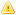Home |Sign in | EnglishHelpWLanguageWLanguage functionsControls, pages and windowsDrawing functionsPrefix syntaxDrawing functions on picLayer variablesDrawPolylinePresentationExampleCoordinatesPattern and PHPDrawing in Browser codeRelated examplesSee alsoAlphaBlendBackgroundChangeModeDrawChordDrawLineDrawPointDrawPolygonDrawPolylineDrawRectangleDrawRectangleGradientDrawRoundedRectangleDrawSliceDrawTextDrawTextRTFEndDrawingFillFontHorizontalSymmetryInvertColorPenPixelColorPixelOpacityResizeRotationStartDrawingVerticalSymmetryThis content has been translated automatically. Click here to view the French version.
• Coordinates
• Pattern and PHP
• Drawing in Browser code
WINDEVWEBDEVWINDEV MobileOthers<picLayer variable>.DrawPolyline (Function)
In french: <Variable picCalque>.DessinePolyligne
Draws an row composed of several segments in an Variable of the picLayer type.
The first coordinate indicates the starting point of the first segment, then each coordinate indicates the end of the previous segment and the beginning of the next segment.
Example
MyWDPicImage is WDPic = "Test.gif"
MyLayer is picLayer

MyLayer.Pen(LightBlue, 2, 4)
arrCoordinatesXY2 is array of 0 int = [2, 2, 140, 2, 150, 70, 0, 100, 200,100]
MyLayer.DrawPolyline(arrCoordinatesXY2)

IMG_MyDrawing = MyWDPicImage
Syntax

Building a line point by point

<picLayer image>.DrawPolyline(<NbPoint> , <X1> , <Y1> , <X2> , <Y2> [, <Xn> [, <Yn> [, <Line color>]]])
<picLayer image>: picLayer variable
Name of the Variable type picLayer to handle.
<NbPoint>: Integer
Number of line segments. This parameter defines the number of <X>, <Y> pairs to specify in the function.
<X1>: Integer
X coordinate of the first segment of the line. These coordinates are expressed in pixels.
<Y1>: Integer
Y coordinate of the first segment of the line. These coordinates are expressed in pixels.
<X2>: Integer
X coordinate of the second segment of the line. These coordinates are expressed in pixels.
<Y2>: Integer
Y coordinate of the second segment of the line. These coordinates are expressed in pixels.
<Xn>: Optional integer
X coordinate of the N segment of the line. These coordinates are expressed in pixels.
<Yn>: Optional integer
Y coordinate of the N segment of the line. These coordinates are expressed in pixels.
<Line color>: Integer or constant (optional)
Line color. This color can correspond to:
If this parameter is not specified, the line color:
• corresponds to the Color specified in the last call to the <Image>.Pen function,
• is identical to the Color used for the Background if the <Image>.Pen function has not been used.

Building a line via an array of X and Y coordinates

<picLayer image>.DrawPolyline(<Array of coordinates> [, <Line color>])
<picLayer image>: picLayer variable
Name of the Variable type picLayer to handle.
<Array of coordinates>: Array of integers or array of points
WLanguage array of integers containing the coordinates of segments that make up the line. The even subscript represent the X coordinates while the odd subscripts represent the Y coordinates of points. These coordinates are expressed in pixels.
<Line color>: Integer or constant (optional)
Line color. This color can correspond to:
If this parameter is not specified, the line color:
• corresponds to the color specified in the last call to <Image>.Pen,
• is identical to the Color used for the Background if the <Image>.Pen function has not been used.Versions 26 and laterConstruction of an row via an Variable type Polygon

<picLayer image>.DrawPolyline(<Polygon> [, <Line color>])
<picLayer image>: picLayer variable
Name of the Variable type picLayer to handle.
<Polygon>: Variable type Polygon
Name of the variable of type Polygon describing the different points of the Polygon.
<Line color>: Integer or constant (optional)
Line color. This color can correspond to:
If this parameter is not specified, the line color:
• corresponds to the color specified in the last call to <Image>.Pen,
• is identical to the Color used for the Background if the <Image>.Pen function has not been used.New in version 26Construction of an row via an Variable type Polygon

<picLayer image>.DrawPolyline(<Polygon> [, <Line color>])
<picLayer image>: picLayer variable
Name of the Variable type picLayer to handle.
<Polygon>: Variable type Polygon
Name of the variable of type Polygon describing the different points of the Polygon.
<Line color>: Integer or constant (optional)
Line color. This color can correspond to:
If this parameter is not specified, the line color:
• corresponds to the color specified in the last call to <Image>.Pen,
• is identical to the Color used for the Background if the <Image>.Pen function has not been used.Construction of an row via an Variable type Polygon

<picLayer image>.DrawPolyline(<Polygon> [, <Line color>])
<picLayer image>: picLayer variable
Name of the Variable type picLayer to handle.
<Polygon>: Variable type Polygon
Name of the variable of type Polygon describing the different points of the Polygon.
<Line color>: Integer or constant (optional)
Line color. This color can correspond to:
If this parameter is not specified, the line color:
• corresponds to the color specified in the last call to <Image>.Pen,
• is identical to the Color used for the Background if the <Image>.Pen function has not been used.
Remarks

Coordinates

Coordinates are given with respect to the top left corner of the image (coordinates: (0.0)).
Related Examples:Unit examples (WINDEV): The drawing functions [ + ] Using the main drawing functions of WINDEV to:- Initialize an Image control for drawing- Draw simple shapes- Write a text into a drawing- Change the color in a drawing
Business / UI classification: Neutral code
Component: wd260pnt.dll
Minimum version required
• Version 25